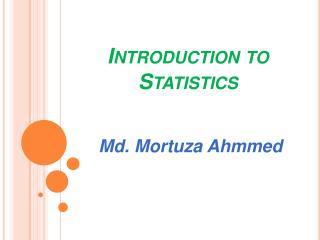DownloadDownload PresentationBasic Statistics Presentation

# Basic Statistics Presentation

Download Presentation## Basic Statistics Presentation

- - - - - - - - - - - - - - - - - - - - - - - - - - - E N D - - - - - - - - - - - - - - - - - - - - - - - - - - -
##### Presentation Transcript

1. Introduction to Statistics Md. MortuzaAhmmed

2. Frequency table

3. simple bar diagram

4. Component bar Diagram

5. Multiple bar Diagram

6. Pie Chart

7. Line graph

8. Histogram

9. Bar diagram vs. histogram

10. Stem and leaf plot

11. Scatter diagram

12. Comparison among the graphs

13. MEASURES OF CENTRAL TENDENCY A measure of central tendency is a single value that attempts to describe a set of data by identifying the central position within that set of data. • Arithmetic mean (AM) • Geometric mean (GM) • Harmonic mean (HM) • Median • Mode

14. Arithmetic mean It is equal to the sum of all the values in the data set divided by the number of values in the data set.

15. Problems • Find the average of the values 5, 9, 12, 4, 5, 14, 19, 16, 3, 5, 7. • The mean weight of three dogs is 38 pounds.  One of the dogs weighs 46 pounds.  The other two dogs, Eddie and Tommy, have the same weight.  Find Tommy’s weight. • On her first 5 math tests, Zany received scores 72, 86, 92, 63, and 77.  What test score she must earn on her sixth test so that her average for all 6 tests will be 80?

16. Affect of extreme values on AM

17. Calculation of AM for grouped data

18. Median

19. Mode

20. when to use the mean, median and mode

21. when we add or multiply each value by same amount

22. mean, median and mode for series data For a series 1, 2, 3 ….n, mean = median = mode = (n + 1) / 2 So, for a series 1, 2, 3 ….100, mean = median = mode = (100 + 1) / 2 = 50.5

23. Geometric mean

24. Harmonic mean

25. AM X HM = (GM) 2

26. Example

27. Example

28. Criteria for good measures of central tendency

29. AM ≥ GM ≥ HM